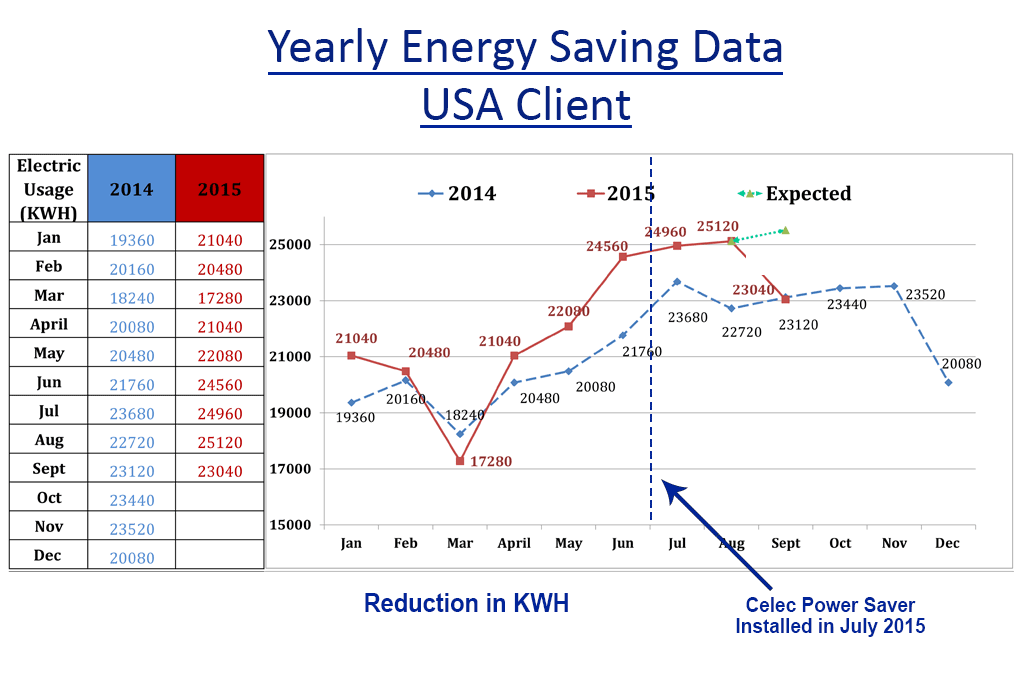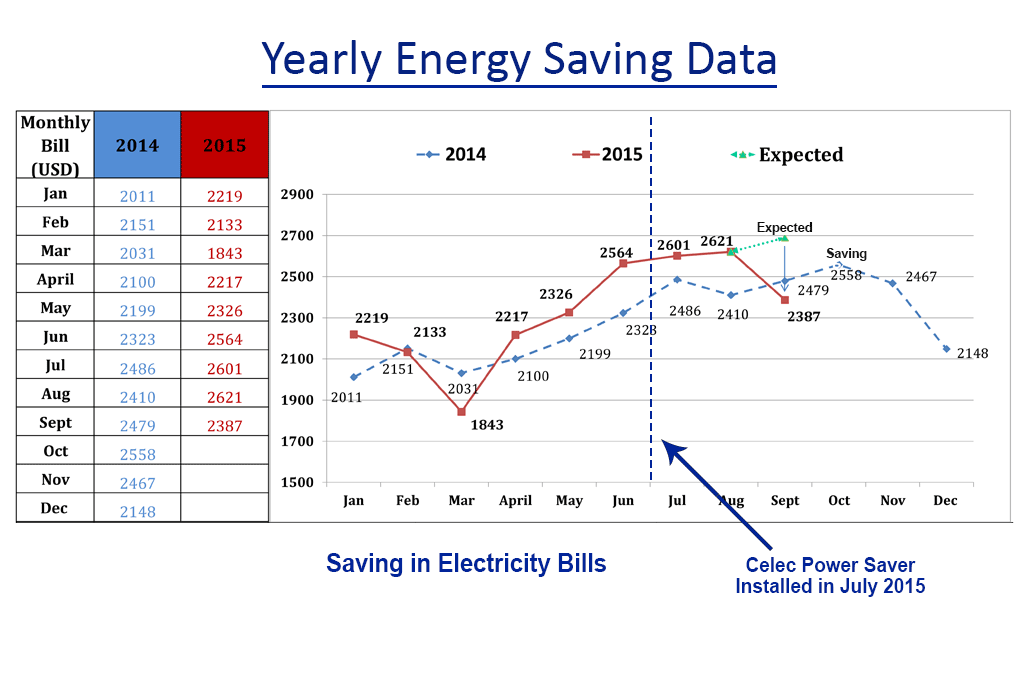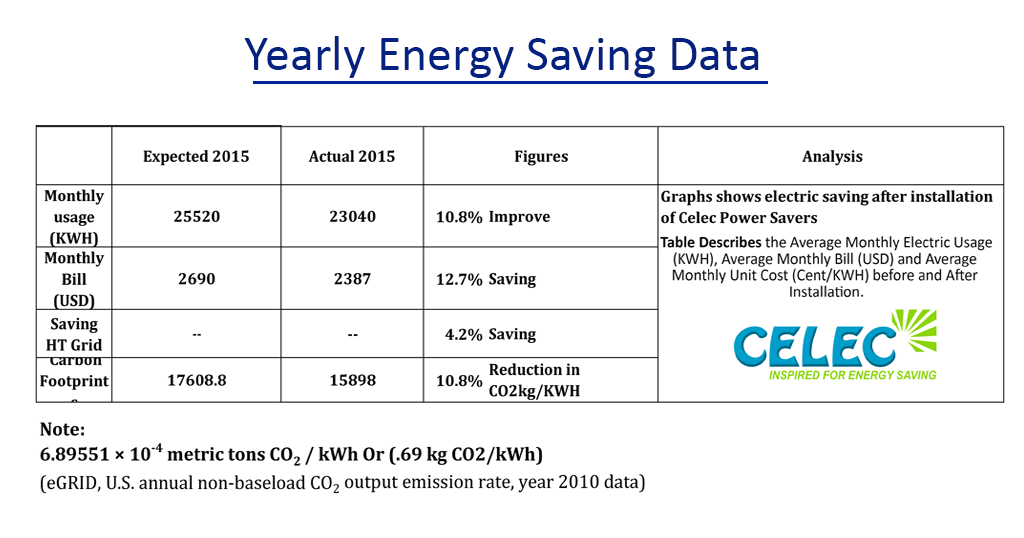# How it Works

• Reduction I2R Losses, improving Power Factor
• Reduction Core Losses in inductive Loads.
• Reduction of electric usage by monitoring the power consumption.
• Reduction of electric breakdown and Surge Suppression
• Reduction in carbon emission.

# Reduced I2R Losses

• Current (Amps) dissipates in the form of Heat in distribution network, Electrical wiring and wire Windings inside transformer and switch gear.
• By Improving power factor current can be reduced to minimum, Accordingly reduction in the losses.
• Note: if the current is reduced to half, losses reduce 4 times as per the following formula.

Power Loss = I2R = Amps2 x Resistance

Thus ampere reduction account for low electricity bills.

# Reduced Core Losses

Electrical Energy is converted to Mechanical power by using magnetic core and alternating current passing  through magnet wire wound  around the core.

When no Current passes through the Magnet wire the internal granules(Dipole) of magnetic core are stationary. CRGO ( Cold Rolled Grain Oriented) .

When AC current passes through the magnet wire the internal grains(Dipole) in the magnetic core rotate as per the alternating current.

There are losses generated like core loss, hysteresis loss, Eddy Current loss in magnetic core and dissipated in the form of  heat. The magnetic core is designed for 50Hz the movement of grains is same as 50Hz voltage waveform.

If any distortion arise in mains voltage waveform, magnetic core respond to distortion by producing extra heat as the grains movement does not change abruptly but tend to move at primary frequency 50Hz. Any distortion in the voltage is converted to heat in core .

For distortion or high frequency on voltage waveform, capacitors provides low impedance path and reduce the  distortion maintain the voltage waveform and reduced core losses achieved.

# Reduced electric usage by monitoring the power consumption

LCD monitor Display shows all electrical Parameters which correspond to power usage. Usually installed near to mains distribution Board so consumer can check usage  and manage the power requirement. Consumers can save another 10-15% just by changing the consumption pattern and habits by monitoring the power consumption.

# Reduced electric breakdown & Surge Suppression

Capacitor bank work as surge suppressor

where energy can be calculated by following formula

Energy = ½ CV2

Energy is joule(J)

Voltage is Volt (V).

Capacitors are tested upto 2000V and maximum short circuit capacity at 10,000Amps.

The minimum capacitor of 5 uf = 10 joules

Energy(J) = ½ x 5 x 10-6 x (2000) = 10 joules

In the model ES-1

capacity range of capacitor bank is from 5 uf to 155 uf

surge suppression capacity is  from 10 joule to 310 joules.

# Reduced Carbon Emission

Power saving lead to Reduced Carbon footprints.

This can be calculated by standard formula for calculation of CO2  emissions from DECC’s organisation.

kWh x Conversion Factor = CO2 in Kgs.

Conversion factor of Electricity is 0.69

For Example, if there is a saving of 1000 units of electricity per month

then carbon emission reduced  1000 x 0.69 = 690 Kg / MonthTHANK YOU

• Reduction I2R Losses, improving Power Factor
• Reduction Core Losses in inductive Loads.
• Reduction of electric usage by monitoring the power consumption.
• Reduction of electric breakdown due to Surge Suppression
• Reduction in carbon emission.

# Reduced I2R Losses

• Current (Amps) dissipates in the form of Heat in distribution network, Electrical wiring and wire Windings inside transformer and switchgear.
• By Improving power factor current can be reduced to minimum, Accordingly reduction in the losses.
• Note: if the current is reduced to half, losses reduce 4 times as per the following formula.

Power Loss = I2R = Amps2 x Resistance

Thus ampere reduction account for low electricity bills.

# Reduced Core Losses

Electrical Energy is converted to Mechanical power by using magnetic core and alternating current passing  through magnet wire wound  around the core.

Example as shown in figure below.When no Current passes through the Magnet wire the internal granules(Dipole) of magnetic core are stationary. CRGO ( cold Rolled Grain Oriented) As shown above.

When AC current passes through the magnet wire the internal grains(Dipole) in the magnetic core rotate as per the alternating current. As shown below.There are losses generated like core loss, hysteresis loss, Eddy Current loss in magnetic core and dissipated in the form of  heat. The magnetic core is designed for 50Hz the movement of grains is same as 50Hz voltage waveform.

If any distortion arise in mains voltage waveform, magnetic core respond to distortion by producing extra heat as the grains movement does not change abruptly but tend to move at primary frequency 50Hz. Any distortion in the voltage is converted to heat in core .

For distortion or high frequency on voltage waveform, capacitors provides low impedance path and reduce the  distortion maintain the voltage waveform and reduced core losses achieved.

# Reduced electric usage by monitoring the power consumption

LCD monitor Display shows all electrical Parameters which correspond to power usage. Usually installed near to mains distribution Board so consumer can check usage  and manage the power requirement. Consumers can save another 10-15% just by changing the consumption pattern and habits by monitoring the power consumption.

# Reduced electric breakdown & Surge Suppression

Capacitor bank work as surge suppressor

where energy can be calculated by following formula

Energy = ½ CV2

Energy is joule(J)

Voltage is Volt (V).

Capacitors are tested upto 2000V and maximum short circuit capacity at 10,000Amps.

The minimum capacitor of 5 uf = 10 joules

Energy(J) = ½ x 5 x 10-6 x (2000) = 10 joules

In the model ES-1

capacity range of capacitor bank is from 5 uf to 155 uf

surge suppression capacity is from 10 joule to 310 joules.

# Reduced Carbon Emission

Power saving lead to Reduced Carbon footprints.

This can be calculated by standard formula for calculation of CO2  emissions from DECC’s organisation.

kWh x Conversion Factor = CO2 in Kgs.

Conversion factor of Electricity is 0.69

For Example, if there is a saving of 1000 units of electricity per month

then carbon emission reduced  1000 x 0.69 = 690 Kg / Month

Yearly Energy Saving Data USA clientYearly Energy Saving Data USAYearly Energy Saving Data USATHANK YOU